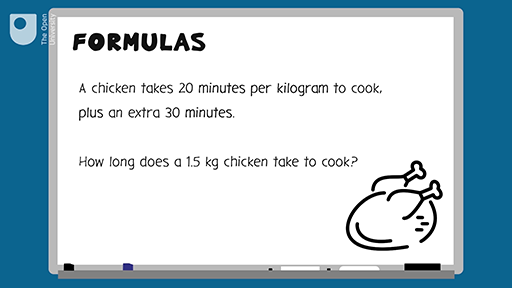Everyday maths 1

Start this free course now. Just create an account and sign in. Enrol and complete the course for a free statement of participation or digital badge if available.

Free course

# 9.1 Formulas in words

You use formulas a lot throughout a normal day, as the examples below show.

## Example: A formula to calculate earnings

Daniel is paid £6.50 per hour. How much does he earn in ten hours?

### Method

You’re told that ‘Daniel is paid £6.50 per hour’.

This is a formula. You can use it to work out how much Daniel earns in a given number of hours. The calculation you need to do is:

Daniel’s pay = £6.50 × number of hours

You’ve been asked how much Daniel earns in ten hours, so put ‘10’ into the calculation in place of ‘number of hours’:

£6.50 × 10 = £65.00

You can use the same formula to work out how much Daniel earns for any number of hours.

You will need to be able to use formulas that have more than one step. The next example looks at a two-step formula.

## Example: A cooking formula

What are the two steps in word formulas? Watch the following video to find out.Skip transcript

#### Transcript

A chicken takes 20 minutes per kilogram to cook, plus an extra 30 minutes. So how long does a 1.5-kilogram chicken take to cook? The formula here is 20 minutes per kilogram, plus 30 minutes.

So to work out how long a 1.5-kilogram chicken takes to cook, you’ll need a formula with two steps.

Step 1: 20 minutes times number of kilograms.

So if you write this out as a formula: 20 times number of kilograms plus 30 equals cooking time. You don’t need to worry about why there are brackets in this formula. That’s how we know that we need to do this sum first before we add the 30 minutes.

Then you just need to put the numbers in the right place. In this case, you would replace number of kilograms with 1.5. 20 times 1.5 plus 30 equals 60 minutes. Your chicken will take one hour to cook.

End transcript

Interactive feature not available in single page view (see it in standard view).

Now test your learning with the following word problems.

## Activity 21: Using formulas

1. Harvey earns £7.75 per hour. How much will Harvey earn in 8 hours?

To answer this you need to multiply the amount Harvey earns in an hour (£7.75) by the number of hours (eight):

• £7.75 × 8 = £62.00
1. A joint of pork takes 40 minutes per kilogram to cook, plus an extra 30 minutes to ensure the outside is crisp.
• a.How long will it take for a 2 kg joint of pork to cook?
• b.How long will it take for a 1.5 kg joint of pork to cook?

• a.You need to use a two-step formula to answer each of these questions. To work out how long a 2 kg joint of pork takes to cook, you’ll need a formula with two steps:

• Step 1: 40 minutes × number of kilograms

Written as a formula, this is:

• (40 × number of kilograms) + 30 = cooking time

So a 2 kg joint would take:

• (40 × 2) + 30 = 110 minutes, or 1 hour and 50 minutes
• b.Using the same formula, a 1.5 kg joint would take:
• (40 × 1.5) + 30 = 90 minutes, or 1 hour and 30 minutes
1. A mobile phone contract costs £15 a month for the first four months, then £20 a month after that. How much will the phone cost for one year?

The information in the question gives you two formulas. To answer the question you need to find the answers to both formulas and add the results together.

The contract costs £15 a month for the first four months. So the formula for this part of the contract is:

• £15 × 4 = £60

After the first four months the contract is £20 a month. The question asks you the total cost of the phone contract for one year, so you need to calculate how much you would pay for another eight months:

• £20 × 8 = £160

So the total cost of the contract for one year is:

• £60 + £160 = £220
FSM_1